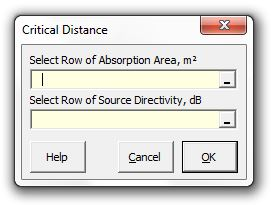### Strutt Help

Critical Distance    1/1,1/3

Strutt|Electroacoustics|Critical Distance calculates the distance from a directional source at which the direct and reverberant sound levels are equal. Select the row of reverberation times and the source directivity from the worksheet and insert the room volume (m3) in the pop-up menu and Strutt will calculate the critical distance and inserts the result into the active worksheet.Strutt implements the formula as:

D_C = 0.057sqrt((QV)/(RT))

Where,
D_C is a critical distance, m
V is the room volume, m3
RT is the reverberation time (s) at given frequency
Q is the directivity of source (eg loudspeaker) at the same frequency